NEET  >  NEET Previous Year Questions (2014-22): Electrostatics Potential & Capacitance

# NEET Previous Year Questions (2014-22): Electrostatics Potential & Capacitance - Notes | Study Physics Class 12 - NEET

 1 Crore+ students have signed up on EduRev. Have you?

Q.1. A capacitor of capacitance C = 900 pF is charged fully by 100 V battery B as shown in figure (a). Then it is disconnected from the battery and connected to another uncharged capacitor of capacitance C = 900 pF as shown in figure (b). The electrostatic energy stored by the system (b) is                   (2022)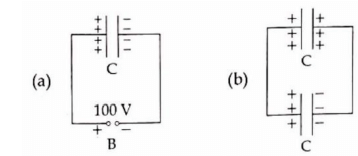(1) 3.25 × 10–6

(2) 2.25 × 10–6

(3) 1.5 × 10–6

(4) 4.5 × 10–6 J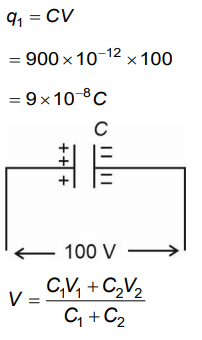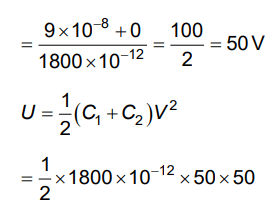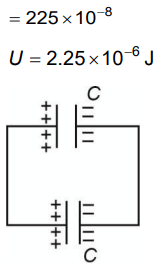Q.2.  Two hollow conducting spheres of radii R1 and R2 (R1 >> R2) have equal charges. The potential would be                 (2022)
(1) More on smaller sphere
(2) Equal on both the spheres
(3) Dependent on the material property of the sphere
(4) More on bigger sphere

Potential of conducting hollow sphere = KQ/R
Now, Q = same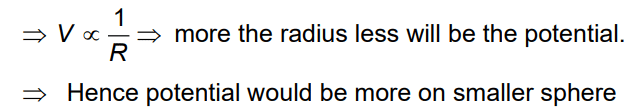Q.3. The angle between the electric lines of force and the equipotential surface is         (2022)
(1) 45°
(2) 90°
(3) 180°
(4) 0°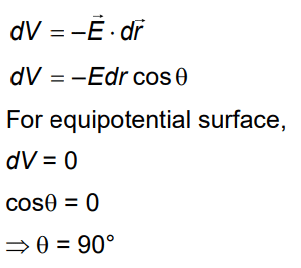Q.4. A parallel plate condenser has a uniform electric field E(V/m) in the space between the plates. If the distance between the plates is d(m) and area of each plate is A(m2), the energy (joule) stored in the condenser is      (2021)
A: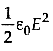B: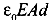C: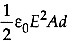D: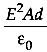Ans: C
Capacitance of a parallel plate capacitor isPotential difference between the plates is
V = Ed      ........ (ii)
The energy stored in the capacitor isQ.5. The equivalent capacitance of the combination shown in the figure is:       (2021)A: C/2
B: 3C/2
C: 3C
D: 2C
Ans:
D(one capacitor gets short)
⇒ Ceq = C1 + C2
= C + C
= 2C

Q.6 Two charged spherical conductors of radius R1 and R2 are connected by a wire. Then the ratio of surface charge densities of the spheres (σ12) is :      (2021)
A: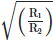B: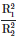C: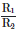D: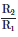Ans: D
For a conducting sphere,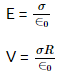As both spheres have same potential after connecting  with wire,
V1 = V2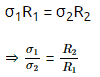Q.7. Twenty seven drops of same size are charged at 220 V each. They combine to form a bigger drop. Calculate the potential of the bigger drop.      (2021)
A: 1520 V
B: 1980 V
C: 660 V
D: 1320 V
Ans:
B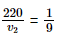⇒ V2 = 220 × 9
= 1980 Volt

Q.8. In a certain region of space with volume 0.2 m3, the electric potential is found to be 5 V throughout. The magnitude of electric field in this region is:      (2020)
A: 1 N/C
B: 5 N/C
C: Zero
D: 0.5 N/C
Ans: C
given v = const. (5 volt)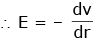E = 0

Q.9. The capacitance of a parallel plate capacitor with air as medium is 6 μF. With the introduction of a dielectric medium, the capacitance becomes 30 μF. The permittivity of the medium is :       (2020)
0 = 8.85×10-12 C2 N-1 m-2
A: 0.44×10-10 C2 N-1 m-2
B: 5.00 C2 N-1 m-2
C: 0.44×10-13 C2 N-1 m-2
D: 1.77×10-12 C2 N-1 m-2
Ans: A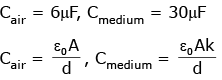Cmed = KCair
30μF = K6μF
K = 5
∴ ε = ε0k = 8.85 × 10-12 × 5
ε = 44.25 × 10-12
ε = 0.4425 × 10-10
ε = 0.44 × 10-10 C2N-1m-2

Q.10. A 40 μF capacitor is connected to a 200 V, 50 Hz ac supply. The rms value of the current in the circuit is, nearly:      (2020)
A: 2.5 A
B: 25.1 A
C: 1.7 A
D: 2.05 A
Ans:
A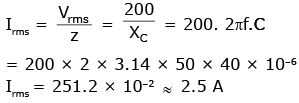Q.11. The electrostatic force between the metal plates of an isolated parallel plate capacitor C having a charge Q and area A, is :-     (2018)
A: independent of the distance between the plates.
B: linearly proportional to the distance between the plates
C: proportional to the square root of the distance between the plates.
D: inversely proportional to the distance between the plates.
Ans:
A
Solution:

F =  QE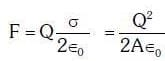∴ Electrostatic force is independent of distance between plates

Q.12. A capacitor is charged by a battery. The battery is removed and another identical uncharged capacitor is connected in parallel. The total electrostatic energy of resulting system :-   (2017)
A: Decreases by a factor of 2
B: Remains the same
C: Increases by a factor of 2
D: Increases by a factor of 4
Ans: A
Solution: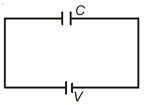Charge on capacitor
q = CV
when it is connected with another uncharged capacitor.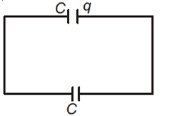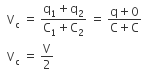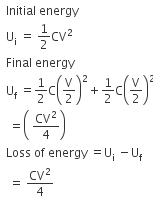Q.13. The diagrams below show regions of equipotentials:-     (2017)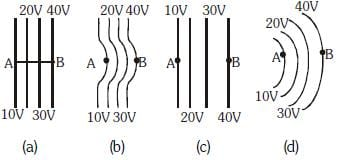A positive charge is moved from A to B in each diagram.
A: Maximum work is required to move q in figure (c)
B: Minimum work is required to move q in figure (a)
C: Maximum work is required to move q in figure (b)
D: In all the four cases the work done is the same
Ans:
D
Solution:
W = qΔV
as ΔV is same in all conditions, work will be same.

Q.14. A capacitor of 21F is charged as shown in the diagram. When the switch S is turned to position 2, the percentage of its stored energy dissipated is :    (2016)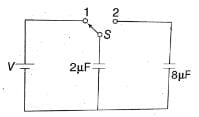A: 80%
B: 0%
C: 20%
D: 75%
Ans:
A
Solution:

Consider the figure given above.
When switch S is connected to point 1, then initial energy stored in the capacitor is given as,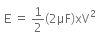When the switch S is connected to point 2, energy dissipated on connection across 8μF will be,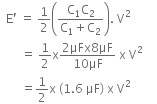Therefore, per centage loss of energy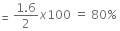Q.15. A parallel plate air capacitor of capacitance C is connected to a cell of emf V and then disconnected from it. A dielectric slab of dielectric constant K, which can just fill the air gap of the capacitor, is now inserted in it. Which of the following is incorrect ?     (2015)
A: The charge on the capacitor is not conserved.
B: The potential difference between the plates decreases K times
C: The energy stored in the capacitor decreases K times

D: the change in energy stored is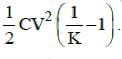Ans:
A
Solution:
A parallel plate air capacitor of capacitance C is connected to a cell of emf V and then disconnected from it.
The charge on the capacitor is given by
Q = CV
The energy stored in the capacitor is
E = 1/2 CV2
When a dielectric slab of dielectric constant K is inserted in it, the charge Q is conserved.
The capacitance becomes K times the original capacitance. (C' = KC)
The voltage becomes 1/K time the original voltage
V' = V/K
The change in energy stored is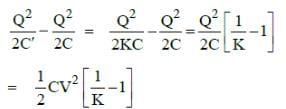Q.16. In a region, the potential is represented by V(x, y, z) = 6x − 8xy − 8y + 6yz, where V is in volts and x, y, z are in meters. The electric force experienced by a charge of 2 coulomb situated at point (1,1,1) is:     (2014)
A: 24N
B: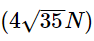C: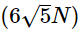D: 30N
Ans:
B
Solution: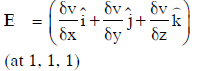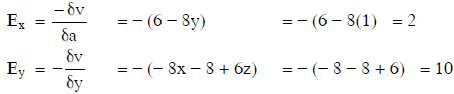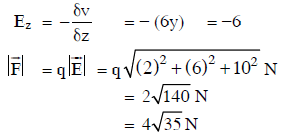Q.17. A conducting sphere of radius R is given a charge Q. The electric potential and the electric field at the centre of the sphere respectively are :    (2014)
A: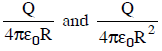B: Both are zero
C: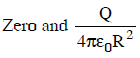D: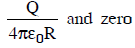Ans:
D
Solution: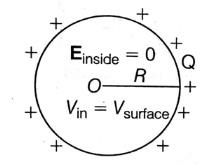Electric field inside, Einside = 0
Potential, Vinside = Vsurface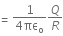The document NEET Previous Year Questions (2014-22): Electrostatics Potential & Capacitance - Notes | Study Physics Class 12 - NEET is a part of the NEET Course Physics Class 12.
All you need of NEET at this link: NEET

## Physics Class 12

157 videos|452 docs|213 tests
 Use Code STAYHOME200 and get INR 200 additional OFF

## Physics Class 12

157 videos|452 docs|213 tests

### How to Prepare for NEET

Read our guide to prepare for NEET which is created by Toppers & the best Teachers

Track your progress, build streaks, highlight & save important lessons and more!

,

,

,

,

,

,

,

,

,

,

,

,

,

,

,

,

,

,

,

,

,

;